# Python | Pandas MultiIndex.nlevels

• Last Updated : 24 Dec, 2018

Python is a great language for doing data analysis, primarily because of the fantastic ecosystem of data-centric python packages. Pandas is one of those packages and makes importing and analyzing data much easier.

Pandas` MultiIndex.nlevels` attribute returns the integer number of levels in the MultiIndex.

Syntax: MultiIndex.nlevels

Example #1: Use `MultiIndex.nlevels` attribute to find the number of levels in the MultiIndex.

 `# importing pandas as pd``import` `pandas as pd`` ` `# Creating the array``array ``=` `[[``1``, ``2``, ``3``], [``'Sharon'``, ``'Nick'``, ``'Bailey'``]]`` ` `# Print the array``print``(array)`

Output :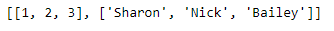Now let’s create the MultiIndex using this array

 `# Creating the MultiIndex``midx ``=` `pd.MultiIndex.from_arrays(array, names ``=``(``'Number'``, ``'Names'``))`` ` `# Print the MultiIndex``print``(midx)`

Output :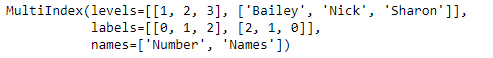Now we will find the number of levels in the MultiIndex.

 `# Print the number of the levels in MultiIndex``midx.nlevels`

Output :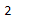As we can see in the output, there are 2 levels in the midx MultiIndex.

Example #2: Use `MultiIndex.nlevels` attribute to find the number of levels in the given MultiIndex.

 `# importing pandas as pd``import` `pandas as pd`` ` `# Creating the array``array ``=` `[[``1``, ``2``, ``3``], [``'Sharon'``, ``'Nick'``, ``'Bailey'``],``            ``[``'Doctor'``, ``'Scientist'``, ``'Physicist'``]]`` ` `# Print the array``print``(array)`

Output :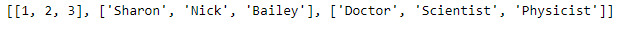Now let’s create the MultiIndex using this array

 `# Creating the MultiIndex``midx ``=` `pd.MultiIndex.from_arrays(array, ``          ``names ``=``(``'Ranking'``, ``'Names'``, ``'Profession'``))`` ` `# Print the MultiIndex``print``(midx)`

Output :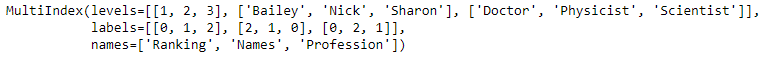Now we will find the number of levels in the MultiIndex.

 `# Print the number of level in MultiIndex``midx.nlevels`

Output :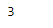As we can see in the output, midx MultiIndex has 3 levels.

My Personal Notes arrow_drop_up